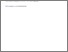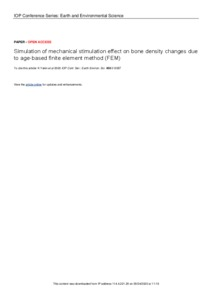# Simulation of mechanical stimulation effect on bone density changes due to age-based finite element method (FEM)

Yakin, Khusnul and Rusmana, Nur Indha and Setyaningsih, Ita and Tirono, Mokhamad (2020) Simulation of mechanical stimulation effect on bone density changes due to age-based finite element method (FEM). Presented at The 10th International Conference on Green Technology (ICGT) "Empowering the Fourth Industrial Revolution through Green Science and Technology, 2-3 Oct 2019, Malang, East Java, Indonesia.Preview
Text
7432.pdf - Published Version

Full text available at: https://iopscience.iop.org/article/10.1088/1755-13...

## Abstract

The getting older of people age, the density of bone will be further reduced. The process speed of bone formation decreases progressively after reaching peak bone mass in the age range 25-30 years. The reduction in bone density is known as osteoporosis. This phenomenon occurs due to an interruption on remodelling bone due to some conditions. Therefore, this study is going to simulate the effect of mechanical stimuli on femur bone density using walking and standing mechanical stimuli. In this study, there are four stages passed. First, build the femur bone construction which consists of two layers (cortical and trabecular). Then input the properties of bone based on age (Young's modulus and Poisson's ratio). Then calculate the stress, strain and strain rate and calculate the bone density using thermodynamic equation of V. Klika and F. Marsik with Runge-Kutta method of 4th orde. The bone density given standing mechanical stimuli (1290 N) for age 25 is 0.7963 g/cm2 and walking mechanical stimuli (1741.5 N) is 1.0698 g/cm2. Then the bone density given standing mechanical stimuli (1200 N) for age 51 is 0.7703 g/cm2 and walking mechanical stimuli (1620 N) is 0.9885 g/cm2.

Item Type: Conference (Paper) 01 MATHEMATICAL SCIENCES > 0103 Numerical and Computational mathematics > 010302 Numerical Solution of Differential and Integral Equations02 PHYSICAL SCIENCES > 0299 Other Physical Sciences > 029901 Biological Physics02 PHYSICAL SCIENCES > 0299 Other Physical Sciences > 029903 Medical Physics01 MATHEMATICAL SCIENCES > 0103 Numerical and Computational mathematics02 PHYSICAL SCIENCES > 0202 Classical Physics Faculty of Mathematics and Sciences > Department of Physics Khusnul Yakin 27 Nov 2020 19:08View Item